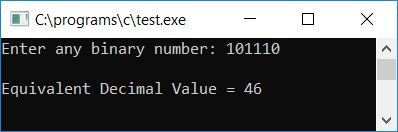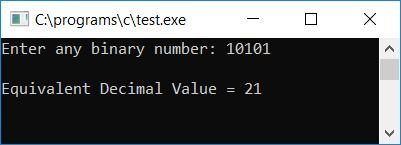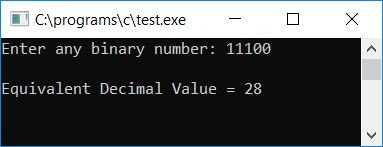# C Program to Convert Binary to Decimal

In this article, we will learn how to create a program in C that converts any given binary number (provided by the user at run-time) to its equivalent decimal value. At last, we have also created a program for the same purpose using a user-defined function named BinToDec().

But before going through the program, if you are not aware of how the binary to decimal conversion takes place, then refer to the step-by-step process of binary to decimal conversion. Now let's move on to the program.

## Binary to Decimal in C

To convert a binary number to a decimal number in C programming, you have to ask the user to enter the binary number and then convert it into a decimal number, as shown in the following program:

```#include<stdio.h>
#include<conio.h>
#include<math.h>
int main()
{
int binnum, decnum=0, i=0, rem;
printf("Enter any binary number: ");
scanf("%d", &binnum);
while(binnum!=0)
{
rem = binnum%10;
decnum = decnum + rem*pow(2,i);
i++;
binnum = binnum/10;
}
printf("\nEquivalent Decimal Value = %d", decnum);
getch();
return 0;
}```

Because the above program was written in the Code::Blocks IDE, you will receive the following output after a successful build and run. This is the first snapshot of the sample run:Now supply any binary number, say 101110, and press the ENTER key to see its equivalent value in the decimal number system, as shown in the second snapshot of the sample run given below:#### Program Explained

• Receive any binary number from the user at program runtime, say 101110.
• Create a while loop that runs until the value of binnum (the binary number entered by the user) becomes 0.
• In other words, the loop continues running until the value of binnum does not equal 0. And when the value of binnum gets equal to 0, then the program flow exits the loop.
• At the first run of the while loop, the condition binnum!=0 or 101110!=0 evaluates to true, therefore program flow goes inside the loop, and binnum%10 or 101110%10 or 0 gets initialized to rem.
• And decnum + rem*pow(2, i) (at the start of the program, decnum and i were both set to 0). And the function pow() of the math.h library takes two arguments; the first argument corresponds to the base, and the second argument corresponds to the exponent. That is, in 23, 2 is the base and 3 is the exponent, so 0 + 0*pow(2, 0), 0 + 0*20, 0 + 0*1, 0+0, or 0 gets initialized to decnum.
• The value of i gets incremented and becomes 1.
• And then binnum/10 or 101110/10 or 10111 gets initialized to binnum.
• Now program flow goes back to the condition of the while loop. That is binnum!=0 or 10111!=0 evaluates to true, therefore program flow again goes inside the loop.
• Inside the loop, binnum%10 or 10111%10 or 1 gets initialized to rem.
• And then decnum + rem*pow(2, i) or 0 + 1*pow(2, 1) or 0 + 1*21 or 2 gets initialized to decnum.
• The value of i gets incremented and becomes 2.
• And binnum/10 or 10111/10 or 1011 gets initialized to binnum.
• Now the program flow goes back to the condition of the while loop again, i.e., the condition binnum!=0 or 1011!=0 evaluates to true, therefore program flow again goes inside the loop.
• Therefore, again, binnum%10 or 1011%10 or 1 gets initialized to rem.
• And then decnum + rem*pow(2, i) or 2 + 1*pow(2, 2) or 2 + 1*22 or 2 + 1*4 or 6 gets initialized to decnum.
• The value of i gets incremented and becomes 3. And then binnum/10 or 1011/10 or 101 gets initialized to binnum.
• In this manner, program flow returns to the condition of the while loop, and if the condition evaluates to true, then repeat the previous steps to calculate the new values of rem, decnum, i, and binnum.
• That is, at the fourth run of the while loop, rem, decnum, i, and binnum hold their values as 1, 14, 4, and 10 respectively.
• Then at the fifth run, rem, decnum, i, and binnum hold 0, 14, 5, and 1 respectively.
• At the end of the sixth run, rem, decnum, i, and binnum have values of 1, 46, 6, and 0, respectively.
• As the value of binnum approaches zero, the program flow does not enter the loop. And we have 46 as the value of decnum, which is the equivalent decimal value of the given binary number, say 101110.
• Now print the value of decnum at the end of the program.

### Binary to Decimal in C without pow() Function

Now let's create the same program, but this time without using any pow() function of math.h library. The question is, Write a program in C that converts any given binary number to decimal number without using any pow() function. The answer to this question is:

```#include<stdio.h>
#include<conio.h>
int main()
{
int binnum, decnum=0, i=1, rem;
printf("Enter any binary number: ");
scanf("%d", &binnum);
while(binnum!=0)
{
rem = binnum%10;
decnum = decnum + (rem*i);
i = i*2;
binnum = binnum/10;
}
printf("\nEquivalent Decimal Value = %d", decnum);
getch();
return 0;
}```

Here is the final snapshot of the sample run of the above program:Here we have replaced pow(2, i) (0 as the initial value of i) with i (1 as the initial value of i) and i++ with i=i*2. Therefore, in this case, we will get:

• Initially, i equals 1.
• In the second run, i equals i*2 or 1*2 or 2.
• In the third run, i equals i*2 or 2*2 or 4.
• i equals i*2 or 4*2 or 8 on the fourth run.
• At the fifth run, i equals i*2 or 8*2 or 16.
• At the seventh run, i equals i*2 or 16*2 or 32.

We obtain the same results as in the case of pow(2, i), namely:

• pow(2, 0) or 20 or 1 on the first run.
• pow(2, 1) or 21 or 2 on the first run.
• pow(2, 2) or 22 or 4 on the first run.
• pow(2, 3) or 23 or 8 on the first run.
• and so on.

### Binary to Decimal in C using a User-Defined Function

Now let's create another program in C that uses a user-defined function named BinToDec() to convert any given binary number into its equivalent decimal value, as shown in the program given below:

```#include<stdio.h>
#include<conio.h>
int BinToDec(int bin);
int main()
{
int binnum, decnum;
printf("Enter any binary number: ");
scanf("%d", &binnum);
decnum = BinToDec(binnum);
printf("\nEquivalent Decimal Value = %d", decnum);
getch();
return 0;
}
int BinToDec(int bin)
{
int dec=0, i=1, rem;
while(bin!=0)
{
rem = bin%10;
dec = dec + (rem*i);
i = i*2;
bin = bin/10;
}
return dec;
}```

Here is the final snapshot of the sample run:Here we have used a function named BinToDec() that takes one argument as a binary number. Inside the function, we have converted the given binary number to a decimal number. The decimal number is stored inside a variable dec. And we have returned the value of dec to the function. Therefore, inside the main() function, we have initialized the returning value of function BinToDec() to a variable decnum that holds the value of dec in the BinToDec() function. Finally, print the value of decnum as output, which will be the equivalent decimal value of the given binary number.

#### The same program in different languages

C Quiz

« Previous Program Next Program »